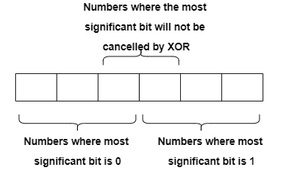GeeksforGeeks App
Open AppBrowser
Continue

# Generate a permutation of [0, N-1] with maximum adjacent XOR which is minimum among other permutations

Given an integer N, the task is to print a permutation of numbers from 0 to N-1, such that:

• There is no duplicate element in the permutation
• The maximum adjacent XOR of this permutation is minimum among other permutations
• There can be more than one permutation present that satisfies these conditions.

Examples:

Input: N = 5
Output: 1 2 3 0 4
Explanation:
The maximum XOR value of two consecutive elements of the permutation is 0 XOR 4 = 4
No other permutation exists where the maximum XOR value of two consecutive elements of the permutation is less than 4.
Other permutations may exist where the value is equal to or greater than 4.

Input: N = 10
Output: 1 2 3 4 5 6 7 0 8 9

Approach: The intuition to solve this problem is based on below mentioned properties of XOR:

• Inside the entire permutation, there will be some numbers with the most significant bit as 1 or as 0.
• So group the numbers with the most significant bit as 1 so the most significant bit gets cancelled out.
• But there will always be a case in the permutation where a number with the most significant bit as 1 will reside before or after a number where the most significant bit is 0. At that point, the most significant bit will not be cancelled by the XOR operation.• In that case, place the minimum number with the most significant bit as 1 and the minimum number with the most significant bit as 0(possibly 0), together to get the maximum XOR value.
• This is the possible minimum value of maximum-XOR value among all permutations.

Follow the steps mentioned below to implement the above observation:

• Initially, calculate the minimum number less than or equal to N-1 having the most significant set bit.
• Print all the numbers less than the minimum number with the most significant set bit starting from 1 consecutively.
• Print 0.
• Print all the numbers starting from a minimum number with a most significant set bit up to N-1

Below is the implementation of the above approach:

## C++

 `// C++ program to implement above approach``#include ``using` `namespace` `std;` `// Function to get the desired permutation``void` `getPermutation(``int` `n)``{``    ``// Calculate the maximum number``    ``// in the permutation``    ``int` `maxnum = n - 1;` `    ``// Calculate the minimum number``    ``// bit is 1 where most significant``    ``int` `num = 1;``    ``while` `(maxnum > 0) {``        ``maxnum /= 2;``        ``num *= 2;``    ``}``    ``num /= 2;` `    ``// Print all numbers less than the number``    ``// where most significant bit is set``    ``for` `(``int` `i = 1; i <= num - 1; i++) {``        ``cout << i << ``" "``;``    ``}` `    ``// Print 0``    ``cout << 0 << ``" "``;` `    ``// Print all the numbers``    ``// greater than or equal to``    ``// the number where``    ``// most significant bit is set``    ``for` `(``int` `i = num; i < n; i++) {``        ``cout << i << ``" "``;``    ``}``}` `// Driver Code``int` `main()``{``    ``int` `N = 5;``  ` `    ``// Function call``    ``getPermutation(N);``    ``return` `0;``}`

## Java

 `// JAVA program to implement above approach``import` `java.util.*;``class` `GFG``{``  ` `    ``// Function to get the desired permutation``    ``public` `static` `void` `getPermutation(``int` `n)``    ``{``      ` `        ``// Calculate the maximum number``        ``// in the permutation``        ``int` `maxnum = n - ``1``;` `        ``// Calculate the minimum number``        ``// bit is 1 where most significant``        ``int` `num = ``1``;``        ``while` `(maxnum > ``0``) {``            ``maxnum /= ``2``;``            ``num *= ``2``;``        ``}``        ``num /= ``2``;` `        ``// Print all numbers less than the number``        ``// where most significant bit is set``        ``for` `(``int` `i = ``1``; i <= num - ``1``; i++) {``            ``System.out.print(i + ``" "``);``        ``}` `        ``// Print 0``        ``System.out.print(``0` `+ ``" "``);` `        ``// Print all the numbers``        ``// greater than or equal to``        ``// the number where``        ``// most significant bit is set``        ``for` `(``int` `i = num; i < n; i++) {``            ``System.out.print(i + ``" "``);``        ``}``    ``}` `    ``// Driver Code``    ``public` `static` `void` `main(String[] args)``    ``{``        ``int` `N = ``5``;` `        ``// Function call``        ``getPermutation(N);``    ``}``}` `// This code is contributed by Taranpreet`

## Python3

 `# Python code for the above approach` `# Function to get the desired permutation``def` `getPermutation(n):` `    ``# Calculate the maximum number``    ``# in the permutation``    ``maxnum ``=` `n ``-` `1` `    ``# Calculate the minimum number``    ``# bit is 1 where most significant``    ``num ``=` `1``    ``while` `(maxnum > ``0``):``        ``maxnum ``=` `maxnum``/``/``2``        ``num ``*``=` `2` `    ``num ``=` `(num``/``/``2``)` `    ``# Print all numbers less than the number``    ``# where most significant bit is set``    ``for` `i ``in` `range``(``1``, num):``        ``print``(i, end``=``" "``)` `    ``# Print 0``    ``print``(``0``, end``=``" "``)` `    ``# Print all the numbers``    ``# greater than or equal to``    ``# the number where``    ``# most significant bit is set``    ``for` `i ``in` `range``(num, n):``        ``print``(i, end``=``" "``)` `# Driver Code``N ``=` `5` `# Function call``getPermutation(N)` `# This code is contributed by Potta Lokesh`

## C#

 `// C# program to implement above approach``using` `System;``class` `GFG``{``  ` `    ``// Function to get the desired permutation``    ``static` `void` `getPermutation(``int` `n)``    ``{``      ` `        ``// Calculate the maximum number``        ``// in the permutation``        ``int` `maxnum = n - 1;` `        ``// Calculate the minimum number``        ``// bit is 1 where most significant``        ``int` `num = 1;``        ``while` `(maxnum > 0) {``            ``maxnum /= 2;``            ``num *= 2;``        ``}``        ``num /= 2;` `        ``// Print all numbers less than the number``        ``// where most significant bit is set``        ``for` `(``int` `i = 1; i <= num - 1; i++) {``            ``Console.Write(i + ``" "``);``        ``}` `        ``// Print 0``        ``Console.Write(0 + ``" "``);` `        ``// Print all the numbers``        ``// greater than or equal to``        ``// the number where``        ``// most significant bit is set``        ``for` `(``int` `i = num; i < n; i++) {``            ``Console.Write(i + ``" "``);``        ``}``    ``}` `    ``// Driver Code``    ``public` `static` `void` `Main()``    ``{``        ``int` `N = 5;` `        ``// Function call``        ``getPermutation(N);``    ``}``}` `// This code is contributed by Samim Hossain Mondal.`

## Javascript

 ``

Output

`1 2 3 0 4 `

Time Complexity: 0(N)
Auxiliary Space: 0(1)

My Personal Notes arrow_drop_up